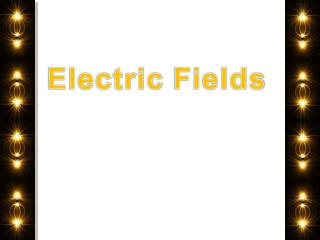DownloadDownload PresentationElectric Fields

# Electric Fields

Download Presentation## Electric Fields

- - - - - - - - - - - - - - - - - - - - - - - - - - - E N D - - - - - - - - - - - - - - - - - - - - - - - - - - -
##### Presentation Transcript

1. ElectricFields

2. What is Electric Field?? Let's take a single electric charge, Q, and put it somewhere. The space around Q is altered – it has the ability to cause a force on any other charge that approaches it. This space contains an electric field. Q

3. What is Electric Field?? Let's take a single electric charge, Q, and put it somewhere. The space around Q is altered – it has the ability to cause a force on any other charge that approaches it. This space contains an electric field. Q What is the value of an electric field? Electric field = Electric force per unit charge

4. What is Electric Field?? Let's take a single electric charge, Q, and put it somewhere. The space around Q is altered – it has the ability to cause a force on any other charge that approaches it. This space contains an electric field. Q What is the value of an electric field? Electric field = Electric force per unit charge q is NOT Q. q is NOT the charge that creates the field! q is a test charge, or second charge brought into the field.

5. Magnitude and Direction of Electric Field The strength (magnitude) of electric fields depend just on distance from the charge Q and on the value of the charge. It does NOT depend on the test charge.

6. Magnitude and Direction of Electric Field The strength (magnitude) of electric fields depend just on distance from the charge Q and on the value of the charge. It does NOT depend on the test charge. E = = = E =

7. Magnitude and Direction of Electric Field The strength (magnitude) of electric fields depend just on distance from the charge Q and on the value of the charge. It does NOT depend on the test charge. E = = = E = Electric field is always in the direction of the force that would occur on a positive test charge.

8. Check for Understanding E = 1) How might you define electric field? Which has a stronger electric field? A -4.0 μC charge or a 2.0 μC charge? 3)The electric field of charge has a value E at 1 m away from the charge. What will be the strength of the electric field at 2 m away from the charge? What is the value of the electric field 0.1 m away from an electron? (HINT: 1 electron has a charge of 1.6 X 10-19 C)

9. Check for Understanding E = • 1) How might you define electric field? • an area around a charge that can exert a force on other charges / the • force per unit charge • Which has a stronger electric field? A -4.0 μC charge or a 2.0 μC charge? • -4.0μCcharge b/c E is proportional to charge • 3)The electric field of a charge has a value E at 1 m away from the charge. What will be the strength of the electric field at 2 m away from the charge? • ¼ E • What is the value of the electric field 0.1 m away from an electron? • (HINT: 1 electron has a charge of 1.6 X 10-19 C) • E = 9109 = 2.91011 N/C 1.610-19 (10-10)2

10. Electric Field Lines Electric field lines show the direction of the force on a positivetest charge Electric field lines point towards negative charges Electric field lines point away from positive charges

11. Electric Field Lines The density of lines shows the relative strength of the electric field This has two implications: larger charges have more field lines radiating 2) As you move farther away from the charge, the strength of the field (density of lines) decreases

12. Net Electric Field Lines If we have two or more charges creating an electric field, we add the vectors from each charge. NOTE: Electric field lines can never cross! That would mean that a test charge would go in two directions at once.

13. Question? • What is the direction of the electric field at point C? • Left • Right • Zero Away from positive charge (right) Towards negative charge (right) y Net E field is to right. C x

14. Question? • What is the direction of the electric field at point A? • Up • Down • Left • Right • Zero A x

15. Question? • What is the direction of the electric field at point B? • Up • Down • Left • Right • Zero y B x

16. Question? • What is the direction of the electric field at point A, if the two positive charges have equal magnitude? • Up • Down • Left • Right • Zero A x